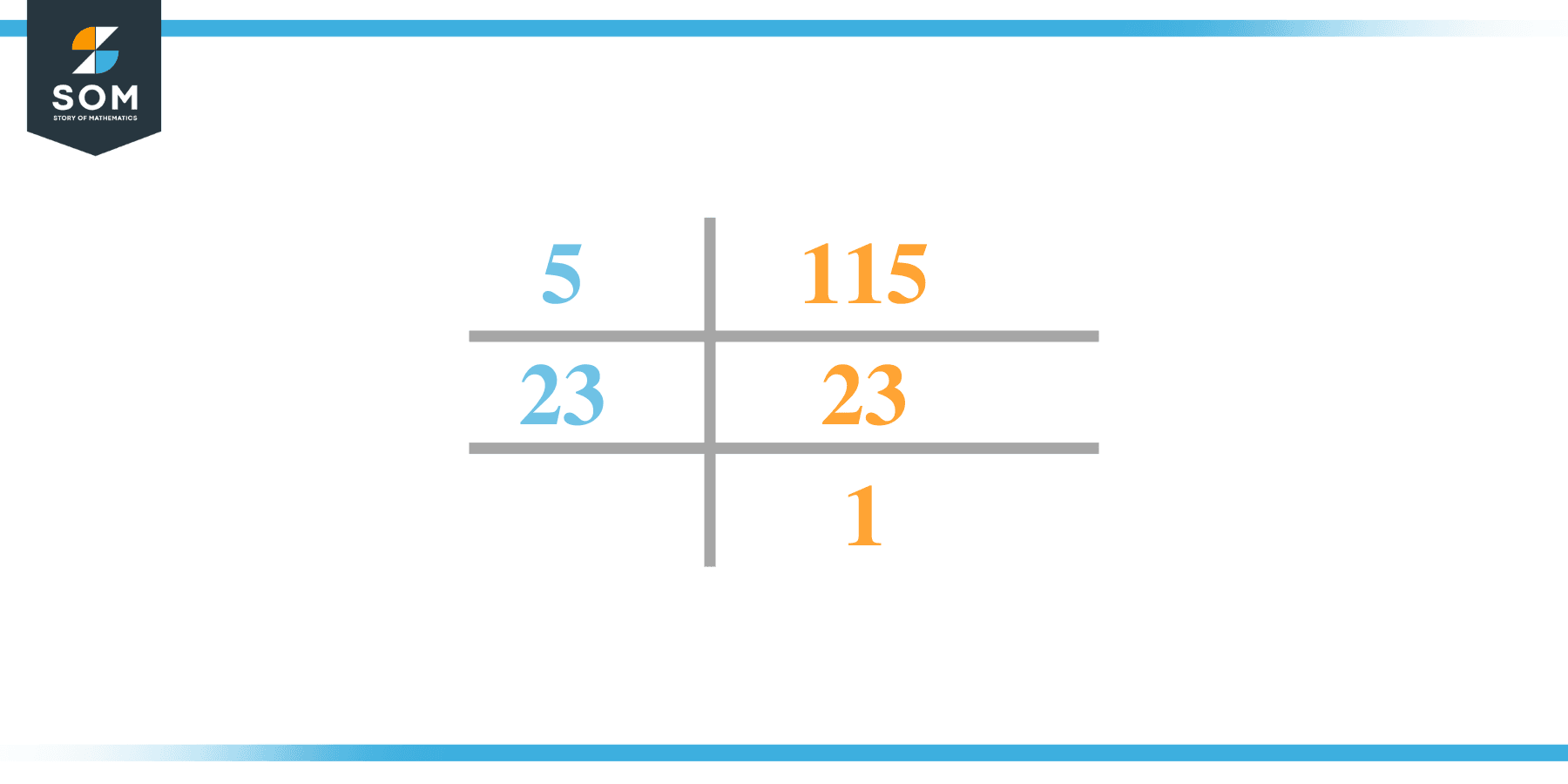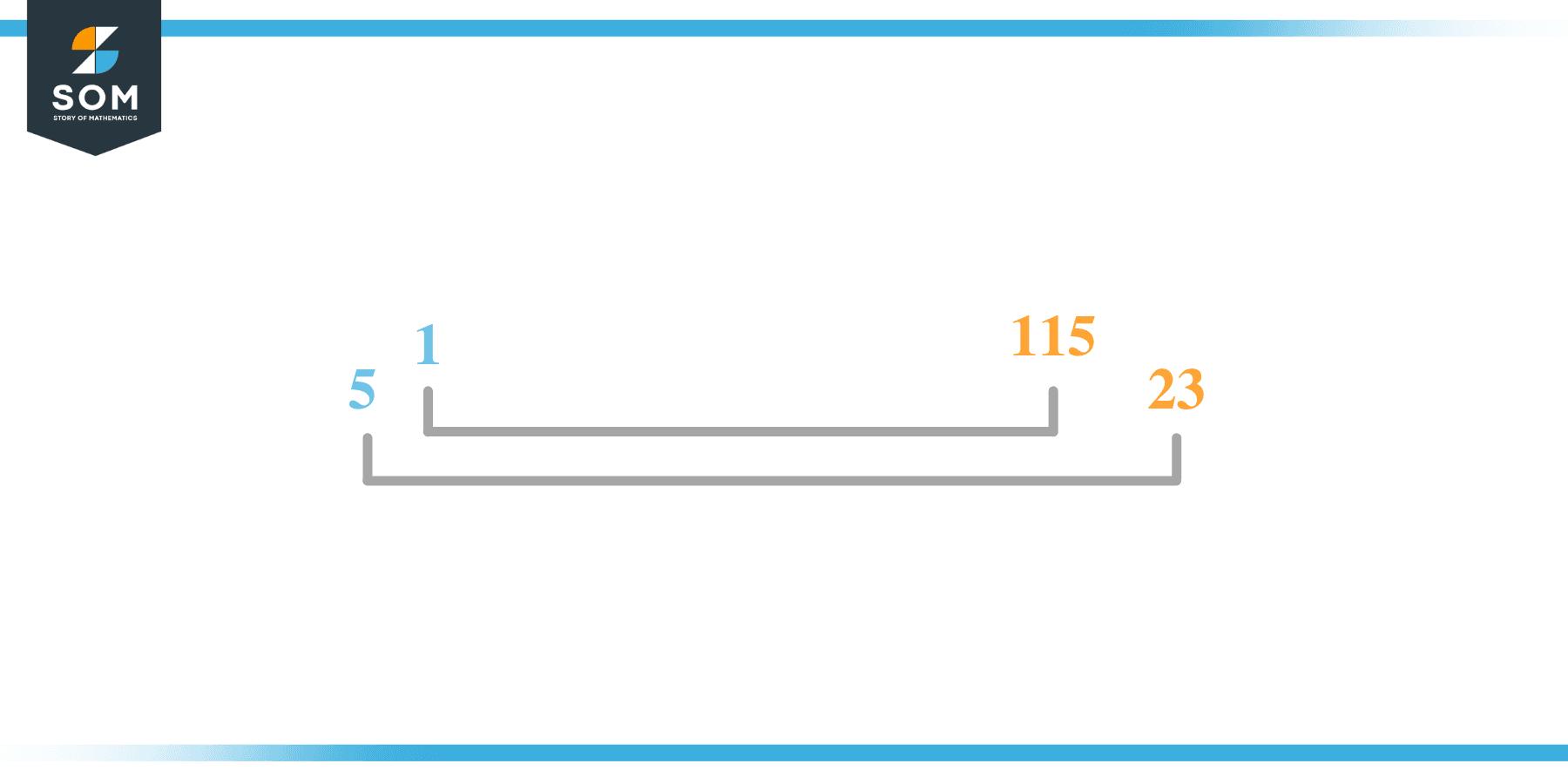# Factors of 115: Prime Factorization, Methods, and Examples

The factors of 115 are the numbers that yield zero as the remainder when 115 is divided by such numbers. These numbers are known as the factors of 115.The number 115 is an odd composite number which means that it has multiple factors. In this article, we will take a look at the various methods through which these factors can be determined.

### Factors of 115

Here are the factors of number 115.

Factors of 115: 1, 5, 23, 115

### Negative Factors of 115

The negative factors of 115 are similar to its positive factors, just with a negative sign.

Negative Factors of 115: -1, -5, -23 and -115

### Prime Factorization of 115

The prime factorization of 115 is the product of its prime factors.

Prime Factorization: 5 x 23

In this article, we will learn about the factors of 115 and how to find them using various techniques such as upside-down division, prime factorization, and factor tree.

## What Are the Factors of 115?

The factors of 115 are 1, 5, 23, and 115. All of these numbers are the factors as they do not leave any remainder when divided by 115.

The factors of 115 are classified as prime numbers and composite numbers. The prime factors of the number 115 can be determined using the technique of prime factorization.

## How To Find the Factors of 115?

You can find the factors of 115 by using the rules of divisibility. The rule of divisibility states that any number when divided by any other natural number then it is said to be divisible by the number if the quotient is the whole number and the resulting remainder is zero.

To find the factors of 115, create a list containing the numbers that are exactly divisible by 115 with zero remainders. One important thing to note is that 1 and 115 are the 115’s factors as every natural number has 1 and the number itself as its factor.

1 is also called the universal factor of every number. The factors of 115 are determined as follows:

$\dfrac{115}{1} = 115$

$\dfrac{115}{5} = 23$

$\dfrac{115}{23} = 5$

$\dfrac{115}{115} = 1$

Therefore, 1, 5, 23, and 115 are the factors of 115.

### Total Number of Factors of 115

For 115 there are 4 positive factors and 4 negative ones. So in total, there are 8 factors of 115.

To find the total number of factors of the given number, follow the procedure mentioned below:

1. Find the factorization of the given number.
2. Demonstrate the prime factorization of the number in the form of exponent form.
3. Add 1 to each of the exponents of the prime factor.
4. Now, multiply the resulting exponents together. This obtained product is equivalent to the total number of factors of the given number.

By following this procedure the total number of factors of 115 is given as:

Factorization of 115 is 1 x 5 x 23.

The exponent of all is 1.

Adding 1 to each and multiplying them together results in 8.

Therefore, the total number of factors of 115 is 8, where 4 are positive factors and 4 are negative factors.

### Important Notes

Here are some important points that must be considered while finding the factors of any given number:

• The factor of any given number must be a whole number.
• The factors of the number cannot be in the form of decimals or fractions.
• Factors can be positive as well as negative.
• Negative factors are the additive inverse of the positive factors of a given number.
• The factor of a number cannot be greater than that number.
• Every even number has 2 as its prime factor which is the smallest prime factor.

## Factors of 115 by Prime FactorizationThe number 115 is composite. Prime factorization is a useful technique for finding the number’s prime factors and expressing the number as the product of its prime factors.

Before finding the factors of 115 using prime factorization, let us find out what prime factors are. Prime factors are the factors of any given number that are only divisible by 1 and themselves.

To start the prime factorization of 115, start dividing by its smallest prime factor. First, determine that the given number is either even or odd. If it is an even number, then 2 will be the smallest prime factor.

Continue splitting the quotient obtained until 1 is received as the quotient. The prime factorization of 115 can be expressed as:

115 = 5 x 23

## Factors of 115 in PairsThe factor pairs are the duplet of numbers that when multiplied together result in the factorized number. Depending upon the total number of factors of the given numbers, factor pairs can be more than one.

For 115, the factor pairs can be found as:

1 x 115 = 115

5 x 23 = 115

The possible factor pairs of 115 are given as (1, 115) and (5, 23).

All these numbers in pairs, when multiplied, give 115 as the product.

The negative factor pairs of 115 are given as:

-1 x -115 = 115

-5 x -23 = 115

It is important to note that in negative factor pairs, the minus sign has been multiplied by the minus sign due to which the resulting product is the original positive number. Therefore, -1, -5, -23, and -115 are called negative factors of 115.

The list of all the factors of 115 including positive as well as negative numbers is given below.

Factor list of 115: 1, -1, 5, -5, 23, -23, 115, and -115

## Factors of 115 Solved Examples

To better understand the concept of factors, let’s solve some examples.

### Example 1

How many factors of 115 are there?

### Solution

The total number of Factors of 115 is 4.

Factors of 115 are 1, 5, 23, and 115.

### Example 2

Find the factors of 115 using prime factorization.

### Solution

The prime factorization of 115 is given as:

115 $\div$ 5 = 23

23 $\div$ 23 = 1

So the prime factorization of 115 can be written as:

5 x 23 = 115